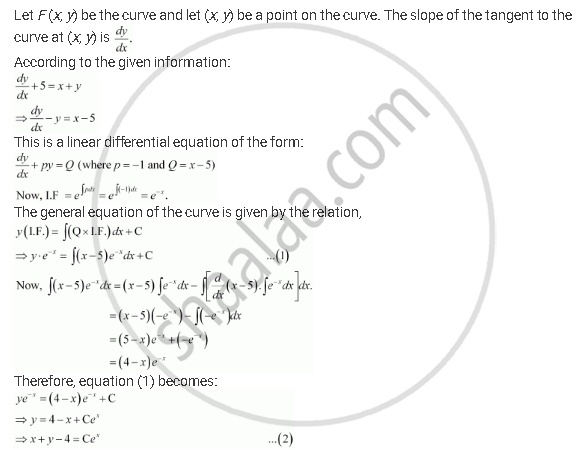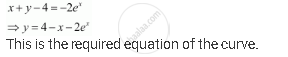Share

# Find the Equation of a Curve Passing Through the Point (0, 2) Given that the Sum of the Coordinates of Any Point on the Curve Exceeds the Magnitude of the Slope of the Tangent to the Curve at that Point by 5. - CBSE (Science) Class 12 - Mathematics

ConceptMethods of Solving First Order, First Degree Differential Equations Linear Differential Equations

#### Question

Find the equation of a curve passing through the point (0, 2) given that the sum of the coordinates of any point on the curve exceeds the magnitude of the slope of the tangent to the curve at that point by 5.

#### SolutionThe curve passes through point (0, 2).

Therefore, equation (2) becomes:

0 + 2 – 4 = Ce0

⇒ – 2 = C

⇒ C = – 2

Substituting C = –2 in equation (2), we get:Is there an error in this question or solution?

#### APPEARS IN

NCERT Solution for Mathematics Textbook for Class 12 (2018 to Current)
Chapter 9: Differential Equations
Q: 17 | Page no. 414

#### Video TutorialsVIEW ALL 

Solution Find the Equation of a Curve Passing Through the Point (0, 2) Given that the Sum of the Coordinates of Any Point on the Curve Exceeds the Magnitude of the Slope of the Tangent to the Curve at that Point by 5. Concept: Methods of Solving First Order, First Degree Differential Equations - Linear Differential Equations.
S